## Recalling Lagrangian Mechanics

The Lagrangian is a function of coordinatesand their time derivatives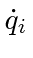.This gives one second order Lagrange equation for each coordinate.

We may define the conjugate momentum to the coordinateasThen Lagrange's equation simply statesThis is one of two Hamilton's equations'' which we will discus in the next section where we will use the momentum rather than the velocity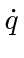to analyze a problem.

Recall that symmetries or invariances give us some conserved quantities in physics. An important example of this is the time independence of the Lagrangian.Of course we think the laws of physics are time independent so this is true in the big picture. We may use it calculate the conserved quantity.chain rule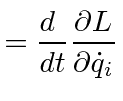Lagrange eq.plug and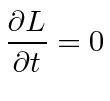sum is total derivativeWe may define the quantity in parentheses to be the Hamiltonian.With this definition, the Hamiltonian is a conserved quantity. (Recall that in relativity, the momentum conjugate to the time coordinate is the total energy.)

Recall that by Euler's theorem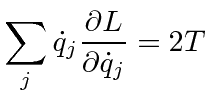ifdoes not depend on the velocities. (This is rather simple.)

So, if the Potential is velocity independent (remember this) then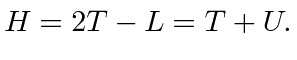This simplifies the calculation of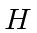for most problems. If the Potential is velocity independent, The Hamiltonian is the total energy and the total energy is conserved if the Lagrangian is time independent.

An important exception to this is Electromagnetism where the magnetic force is velocity dependent and hence the Hamiltonian is not simply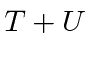, however, it does represent the total energy including the energy in the EM field. The Hamiltonian for an electron in an electromagnetic field is.Jim Branson 2012-10-21